# Go Operators

In this tutorial you will learn about the Go Operators and its application with practical example.

## Go Operators

An operator is a special symbol that is used to carry out some specific operation on its operand. In Go Programming, we have rich set of built in operators to carry out different type of operations. There are operators for assignment, arithmetic operations, logical operations and comparison operations etc. Go Programming operators can be used with many types of variables or constants, but some of the operators are restricted to work on specific data types. Most operators are binary, meaning they take two operands, but a few are unary and only take one operand.

## Type of operators in Go Programming

Go supports the following types of operators –

• Arithmetic Operators
• Assignment Operators
• Comparison (Relational) Operators
• Logical Operators
• Bitwise Operators

## Go Arithmetic Operators

Arithmetic Operators are used to perform arithmetic operations like addition, subtraction, multiplication, division, %modulus, exponent, etc.

Let variable a holds 20 and variable b holds 10, then −

Go Arithmetic operators
Operator Name Description Example
`+` `Addition` ```Addition of given operands ``` `a+b returns 30`
`-` `Subtraction` `Subtraction of second operand from first` ```a-b returns 10 ```
`*` `Multiply` `Multiplication of given operands` ```a*b returns 200 ```
`/` `Division` ```Returns Quotient after division ``` ```a/b returns 2 ```
`%` `Modulus` `Returns Remainder after division` ```a%b returns 0 ```

Example:-

Output:-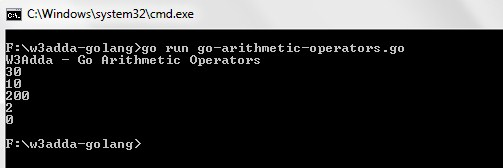## Go Assignment Operators

Assignment operators are used to assign value to a variable, you can assign a variable value or the result of an arithmetical expression.

Go Assignment operators
Operator Description Expression
`=` ```Assignment Operator ``` ```a=b ```
`+=` `add and assign` ```a+=b is equivalent to a=a+b ```
`-=` `subtract and assign` ```a-=b is equivalent to a=a-b ```
`*=` `multiply and assign` ```a*=b is equivalent to a=a*b ```
`/=` `divide and assign` ```a/=b is equivalent to a=a/b ```
`%=` `mod and assign` ```a%=b is equivalent to a=a%b ```
`<<=` `Left shift AND assign` ```a<<=5 is equivalent to a=a<<5 ```
`>>=` `Right shift AND assign` ```a>>=5 is equivalent to a=a>>5 ```
`&=` `Bitwise AND assign` ```a&=5 is equivalent to a=a&5 ```
`^=` `Bitwise exclusive OR and assign` ```a^=5 is equivalent to a=a^5 ```
`|=` `Bitwise inclusive OR and assign` ```a|=5 is equivalent to a=a|5 ```

Example:-

Output:-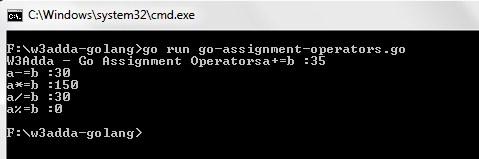## Go Comparison (Relational) Operators

Comparison Operators are used evaluate a comparison between two operands. The result of a comparison operation is a Boolean value that can only be true or false. Comparison Operators are also referred as relational operators.

Let variable a holds 20 and variable b holds 10, then −

Go Relational operators
Operator Description Example
`>` `greater than` ```a>b returns TRUE ```
`<` `Less than` ```a<b returns FALSE ```
`>=` `greater than or equal to` ```a>=b returns TRUE ```
`<=` `less than or equal to` ```a<=b returns FALSE ```
`==` `is equal to` ```a==b returns FALSE ```
`!=` `not equal to` ```a!=b returns TRUE ```

Example:-

Output:-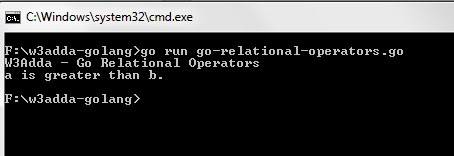## Go Logical Operators

Logical operators are used to combine expressions with conditional statements using logical (AND,OR,NOT) operators, which results in true or false.

Let variable a holds true or 1 and variable b holds false or 0, then −

Go Logical operators
Operator Name Description Example
`&&` `Logical AND` `return true if all expression are true` ```(a && b) returns false ```
`||` `Logical OR` `return true if any expression is true` ```(a || b) returns true ```
`!` `Logical NOT` `return complement of expression` ```!a returns false ```

Example:-

Output:-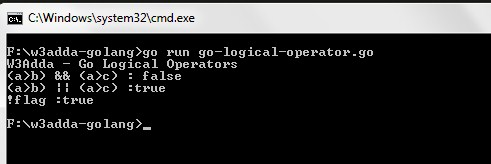## Go Bitwise Operators

Bitwise operator are used to perform bit level operation over its operand.The bitwise logical and shift operators are only applicable to integers.

Go bitwise operators
Operator Description
`&` `bitwise AND`
`|` `bitwise OR `
`^` `bitwise XOR`
`&^` `bit clear (AND NOT)`

Example:-

Output:-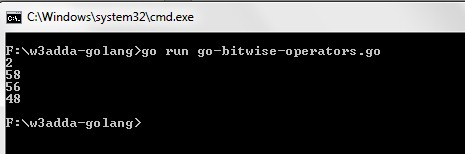## Other Operators

Other Miscellaneous operators in Go
Operator Name Description
`&` `Address of` `&a generates a pointer to a`
`*` `Pointer to` `*a denotes the variable pointed to by a`
`<-` `Receive Operator` <-ch is the value received from channel ch

## String concatenation Operator

In Go, strings can be concatenated using the `+` operator or the `+=` assignment operator.

In this tutorial we have learn about the Go Operators and its application with practical example. I hope you will like this tutorial.